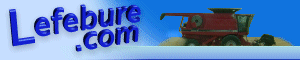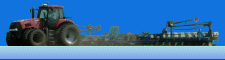Lefebure.com / Articles / NMEA GGA Sentences

Introduction: The NMEA GGA sentence is one of the most common sentences used with GPS receivers. It contains information about position, elevation, time, number of satellites used, fix type, and correction age. Below is an example of a GGA sentence with the data part highlighted in green.

\$GPGGA,115739.00,4158.8441367,N,09147.4416929,W,4,13,0.9,255.747,M,-32.00,M,01,0000*6E

Splitting the data: When processing the sentence, the first thing you want to do is split the sentence into pieces by looking for the comma delimiter. Some example code to do this in VB.net is:

``` Dim pieces() As String = Split(sentence, ",") ```

For the above sentence, youĺll end up with the following items:

115739.00 = Time the position was recorded, in this case 11:57:39.00 UTC. This is always 6 digits, with an optional decimal place and one or two digits after the decimal for receivers with outputs faster than 1 Hz. The output has two digits for hours, two digits for minutes, and two digits for seconds. It can range from 000000 to 235959. The time is always in UTC, regardless of which time zone youĺre in.

4158.8441367 = Latitude in Degrees Minutes. This is a minimum of 4 digits, a decimal, and 2 more digits, but has the option to have additional digits after the decimal place. Of the 4 digits before the decimal, the first two are Degrees, the next two are Minutes. For this example, 41░ 58.8441367 Minutes.

N = North or South Hemisphere. This is always either ĹNĺ or ĹSĺ. When converting to decimal degrees, the southern hemisphere is a negative number.

09147.4416929 = Longitude in Degrees Minutes. This is a minimum of 5 digits, a decimal, and 2 more digits, but has the option to have additional digits after the decimal place. Of the 5 digits before the decimal, the first three are Degrees, the next two are Minutes. For this example, 91░ 47.4416929 Minutes.

W = East or West Hemisphere. This is always either ĹEĺ or ĹWĺ. When converting to decimal degrees, the western hemisphere is a negative number.

4 = Fix type. This is always a single number. Reportable solutions include:
0 = Invalid, no position available.
1 = Autonomous GPS fix, no correction data used.
2 = DGPS fix, using a local DGPS base station or correction service such as WAAS or EGNOS.
3 = PPS fix, Iĺve never seen this used.
4 = RTK fix, high accuracy Real Time Kinematic.
5 = RTK Float, better than DGPS, but not quite RTK.
6 = Estimated fix (dead reckoning).
7 = Manual input mode.
8 = Simulation mode.
9 = WAAS fix (not NMEA standard, but NovAtel receivers report this instead of a 2).

13 = Number of satellites in use. For GPS-only receivers, this will usually range from 4-12. For receivers that support additional constellations such as GLONASS, this number can be 21 or more.

0.9 = Horizontal Dilution of Precision (HDOP). This is a unitless number indicating how accurate the horizontal position is. Lower is better.

255.747 = Elevation or Altitude in Meters above mean sea level.

M = This is always ĹMĺ, presumably for meters, the unit of the previous field.

-32.00 = Height of the Geoid (mean sea level) above the Ellipsoid, in Meters.

M = This is always ĹMĺ, presumably for meters, the unit of the previous field.

01 = Age of correction data for DGPS and RTK solutions, in Seconds. Some receivers output this rounded to the nearest second, some will also include tenths of a second.

0000 = Correction station ID number, used to help you determine which base station youĺre using. This is almost always 4 digits.

Last updated: May 10, 2020